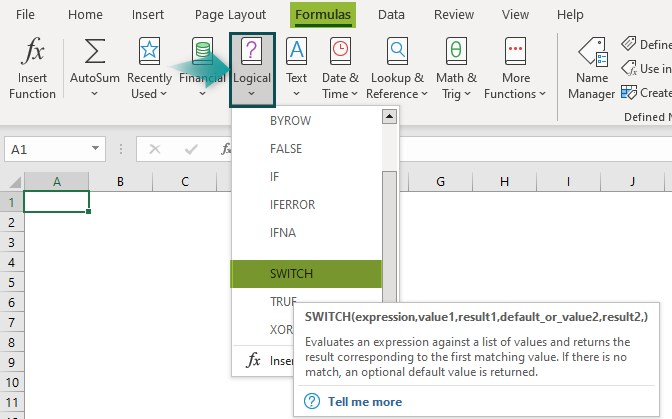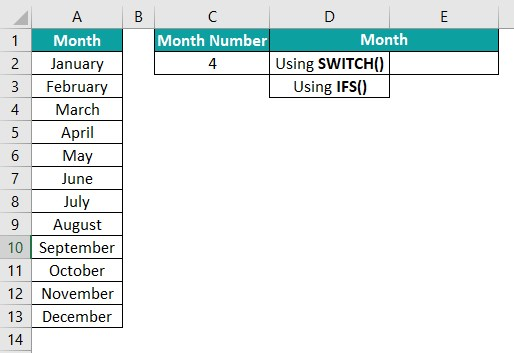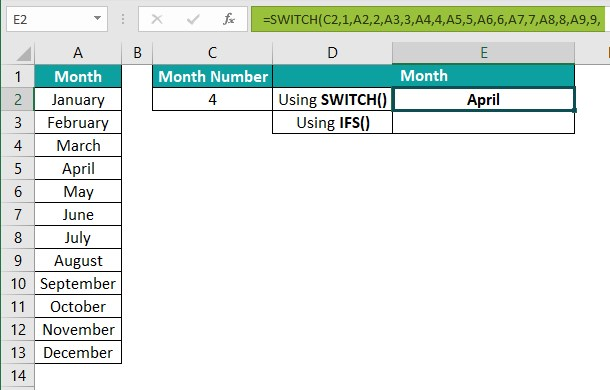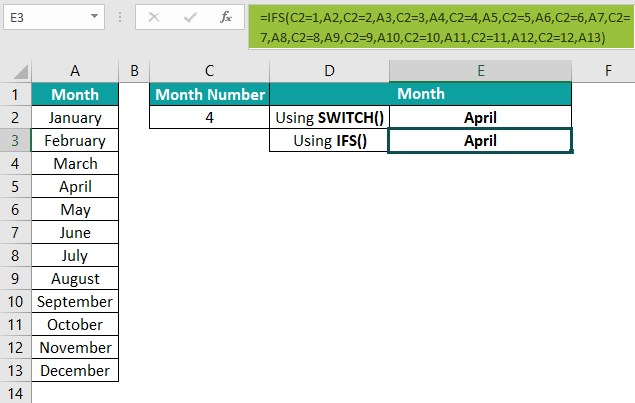# SWITCH Excel FunctionArticle byExcelMojo Team## What Is SWITCH Function In Excel?

The SWITCH function in Excel is a Logical function. It assesses a given value against a range of values to return the output corresponding to the first found match. And in the case of no matching value, the function returns the specified default value.

The SWITCH function is available in Excel 2016 and above. Users can use this function as an effective replacement for nested IF(). For example, the first table in the below image contains a list of grocery items and their corresponding category IDs.

And suppose we require to update each item’s category in column C, based on the legend provided in the range F3:G7. Then, we can apply the SWITCH() in the target cells to populate the required data.

In the above example, the SWITCH Excel function return value in each cell in column C corresponds to the first match found for the respective Category ID (column B).

For instance, in row 4, the category ID in cell B4 is 5. So, the SWITCH() checks for the first match for the value 5, which is Pulses. Thus, the SWITCH Excel function return value in the target cell C4 is Pulses.

###### Key Takeaways
• The SWITCH Excel function checks the supplied value against a list of values and returns the result corresponding to the first match found. Users can apply this function instead of nested IF, as it reduces the formula length and complexity while allowing one to test more than one condition.
• The SWITCH() takes the arguments expression, value1…..value126, result1…..result126, and default as input. While the arguments expression, value1, and result1 are mandatory, the remaining arguments are optional.
• The SWITCH() works only for scenarios where we must determine an exact match for a given value. But it also yields excellent results when used with other inbuilt Excel functions such as MONTH and RIGHT.

### SWITCH() Excel Formula

The SWITCH Excel formula syntax is:

where,

• expression: The value the SWITCH Excel formula would compare against value1…..value126.
• value1: The value the function compares against the expression.
• result1: The value the SWITCH() should return when the corresponding valueN matches the expression.
• default_or_value2,result2: The value the SWITCH() should return when there is no match in the valueN expressions.

While the first three SWITCH function arguments are mandatory, the default argument is optional.

Meanwhile, SWITCH function may not work due to the following reasons:

• The argument expression can be a number, text value, Excel cell reference, named range, Boolean value, function, or formula.
• We should provide the resultN argument for every corresponding valueN argument supplied.
• We can enter a maximum of 126 pairs of valueN and resultN as SWITCH Excel function arguments since Excel functions typically accept 254 arguments.
• If the SWITCH() does not detect a match for the given expression and there is no default value, the function throws the #N/A error.

The above explanation for the SWITCH() arguments might appear complicated. But the SWITCH examples in the subsequent sections will help us understand them clearly.

### How To Use Switch Excel Function?

Use the below steps to apply the SWITCH Excel function.

1. Ensure the values we need to match for are numbers, text values, cell references, named ranges, Boolean values, functions, or formulas.

2. Then, select the target cell and enter the SWITCH().

3. Finally, press Enter to view the SWITCH() output.

Here is an example to explain the above steps in detail.

The following table shows employees’ performance ratings on a scale of 1 to 5.

And suppose we have to update each employee rating in column C based on the corresponding rating value in column B. Then, here is how we can apply the SWITCH() in the target cells to get the required data.

Step 1: Select the target cell C2, enter the following SWITCH(), and press Enter.

=SWITCH(B2,1,”Poor Performance”,2,”Needs Improvement”,3,”Meets Expectations”,4,”Exceeds Requirements”,5,”Exceptional Work”,”No Rating”)

We can also enter the rating number directly as the expression argument. For example, the SWITCH() in the target cell C2 can be:

=SWITCH(3,1,”Poor Performance”,2,”Needs Improvement”,3,”Meets Expectations”,4,”Exceeds Requirements”,5,”Exceptional Work”,”No Rating”)

Alternatively, we can access the function from the Formulas tab. And for that, we need to select the target cell C2 and click FormulasLogicalSWITCH.

Once we select the SWITCH(), the Function Arguments window will open, showing two fields, as depicted below.

Enter the two field values, and once we click on the Value1 field, the Result1 field will appear, where we can enter the corresponding value. Then, click on the Result1 field to get the next field, Default_or_value2. Next, when we click on the Default_or_value2 field, the window will have 126 pairs of fields to enter the valueN and resultN values.

Also, please note that the last argument we enter will be the default argument. And in this case, it is No Rating. And if we do not have a default value, we can stop entering the arguments with the last result field.

And once we click OK in the Function Arguments window, the SWITCH() will get executed.

Thus, entering the arguments in the above-explained ways will ensure that SWITCH Excel function works correctly.

Step 2: Drag the fill handle downwards to copy the formula in the range C3:C11.

Let us consider the target cell C11 formula to understand how the SWITCH() works. First, the expression argument is a cell reference to the value 1, B11. Then, the function tries to determine the matching result for the value 1. In this example, it finds the first match, which is Poor Performance. And thus, it returns Poor Performance as the function output.

### Examples

Here are a few more SWITCH Excel function examples to see how we can use the function effectively.

#### Example #1

This example shows how we can apply the SWITCH Excel function with a logical operator. Consider the below table. It shows the aggregates of ten students.

Suppose the requirement is to enter each student’s grade in column C based on their aggregate. Then, we can use the SWITCH Excel function, with the logical operator, >=, in the target cells and get the required grades.

Step 1: Select the target cell C2, enter the following SWITCH(), and press Enter.

=SWITCH(TRUE,B2>=90,”A”,B2>=80,”B”,B2>=70,”C”,B2>=60,”D”,”Fail”)

Here, the valueN arguments are expressions containing logical operators. Thus, the argument expression provided in the above SWITCH() is TRUE. The function determines the first match for which the aggregate value satisfies the condition. For example, in cell C2, the function finds the cell B2 value, 98, satisfies the first condition or value1. And so, it returns grade A as the output.

Step 2: Drag the fill handle downwards to copy the formula in the range C3:C11.

In the case of the target cell C11, the SWITCH() does not find a match for the given aggregate. And thus, it returns the default value, Fail, as the function output.

#### Example #2

We can apply the SWITCH Excel function with other inbuilt functions, such as MONTH, to achieve excellent results.

Suppose the following table lists the team meeting schedules for the current year. And we have to determine in which quarter each scheduled meeting falls to display the data in the target cell range C2:C11.

Then, here is how we can utilize the SWITCH with the MONTH Excel function.

Step 1: Select the target cell C2, enter the formula provided in the Formula Bar in the below image, and press Enter.

The SWITCH() does not contain the default argument value in this example.

Step 2: Drag the fill handle downwards to copy the formula in the range C3:C11.

Consider the target cell C11 formula. First, the MONTH() returns the month of the date 12/13/2022 as a number 12. Then the SWITCH() checks for a match for the value 12, which is Q4. And thus, we can see Q4 as the function output.

#### Example #3

This example shows how we can apply the SWITCH with RIGHT Excel function to find a match for a part of a given expression.

Assume that column A in the below table shows the US state capitals and state names in the abbreviated form.

And we have to display the full name of the corresponding state against each data point in column B. We can get the required data using the SWITCH Excel function with the RIGHT().

Step 1: Select the target cell B2, enter the below formula containing the RIGHT() within the SWITCH(), and press Enter.

Step 2: Drag the fill handle downwards to copy the formula in the range B3:B8.

Let us see the target cell B8 formula to understand how it works. First, the RIGHT() returns the last two characters from the text in cell A8, AR. And then, the SWITCH() checks for a match for the characters AR. As per the formula, the corresponding resultN argument for the valueN, AR, is Arkansas. So, the SWITCH() returns the value Arkansas as the output.

### Important Things To Note

• The SWITCH Excel function can accept a maximum of 126 pairs of valueN and resultN arguments.
• We should ensure that the argument expression in the SWITCH() is a number, text value, cell reference, named range, Boolean value, function, or formula.
• For every valueN argument supplied to the SWITCH(), there should be a corresponding resultN argument.
• When the SWITCH excel function cannot find a match for the supplied expression, and there is no default value, the function throws a #N/A error.

1. Is there a SWITCH function in Excel?

Yes, Excel has SWITCH function. It is available in the Formulas tab. We can click FormulasLogicalSWITCH to use it.2. Why is the SWITCH() in Excel not working?

The SWITCH() in Excel is not working, perhaps because of the following reasons:
• The SWITCH() argument expression is not a number, text value, cell reference, named range, Boolean value, function, or formula.
• You did not supply a corresponding resultN value for every valueN argument provided to the SWITCH().
• The SWITCH() did not find a match for the given value, and you did not supply the default argument.

3. How is SWITCH Excel function different from IFS?

The SWITCH Excel function is different from IFS() in the following ways:
• The argument expression in the SWITCH() cannot contain logical operators and can appear only once as an argument. On the other hand, the logical_test argument (the equivalent of expression) in IFS() can contain logical operators, and the function can have more than one logical_test argument.
• The SWITCH() is easier to create and less complex than the IFS().
• The formula created using the SWITCH() will be less lengthy than the one created using IFS().

For example, the first table in the below image shows the list of months in a year.And we need to enter the month name corresponding to the given month number in cell C2.
Let us see how to apply the SWITCH() and IFS() to determine the required data.

Step 1: Select the target cell E2, enter the following SWITCH(), and press Enter.

=SWITCH(C2,1,A2,2,A3,3,A4,4,A5,5,A6,6,A7,7,A8,8,A9,9,A10,10,A11,11,A12,12,A13)Step 2: Select the target cell E3, enter the following IFS() provided in Formula Bar in the below image, and press Enter.The above example shows that we cannot use logical operators in the argument expression in the SWITCH(). However, the SWITCH() formula is more straightforward and compact than the IFS().